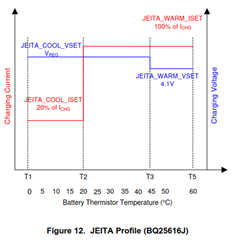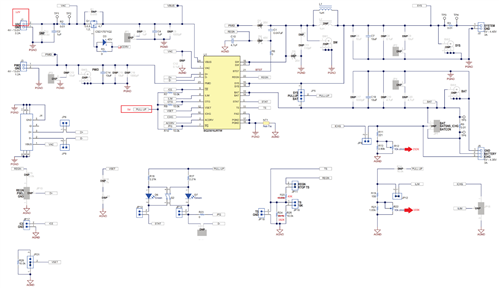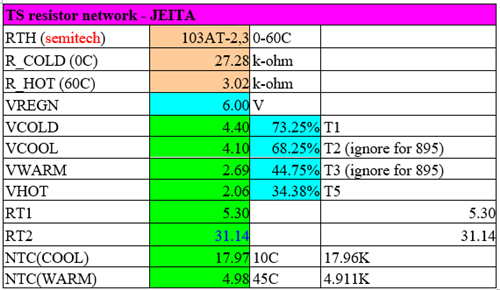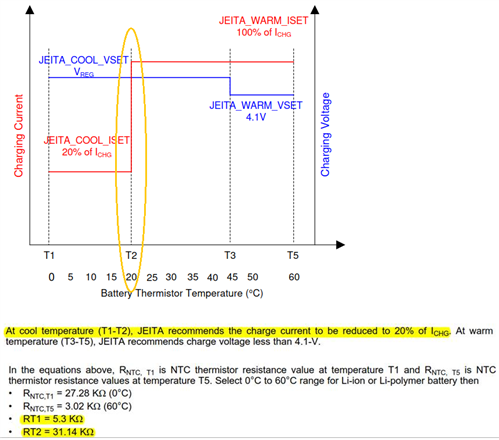If you have a related question, please click the "Ask a related question" button in the top right corner. The newly created question will be automatically linked to this question.

# BQ25616JEVM: JEITA

Part Number: BQ25616JEVM

Dear team

The attachment is the BQ25616J hardware setting,

R12, R20, R24, R22 have to modify the resistance and adjust the value of the variable resistance group,
JP11, JP12 are shorted, JP21 is grounded.

How to calculate the T1.Many thanks

Denny

• Denny,

As described in 9.3.7.4.1 JEITA Guideline Compliance During Charging Mode (BQ25616J), the user defines T1 and T5 then calculate RT1 and RT2.

Thanks,

Ning.

• Hi Ning

The customer use the calculation table provided by calculating tools, the RT1=5.3K, RT2=31.14K you wrote on the spec,
The calculated value is the JEITA interval value of 10 degrees to 45 degrees,
It is not the JEITA interval value of 20 degrees to 45 degrees,

Could you help to check the value of  T2 and T3 from Figure 12.Many thanks

Denny

• Denny,

T2 and T3 should be 10C and 45C respectively.

Thanks,

Ning.